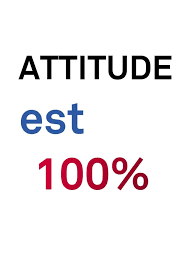Sign in# Le saviez-vous seul votre attitude a un succès de 100% ?Le saviez-vous seul votre Attitude a un succès de 100% ???

J’ai découvert et je veux le partager avec vous.

Si : A, B, C, D, E, F, G, H, I, J, K, L, M, N, O, P, Q, R, S, T, U, V, w, X, Y, Z,

équivalent à : 1, 2, 3, 4, 5, 6, 7, 8, 9, 10, 11, 12, 13, 14, 15, 16, 17, 18, 19, 20, 21, 22 , 23, 24, 25, 26, Alors : TRAVAIL = 83%

T + R + A + V + A + | + L = 20 + 18 + 1 + 22 + 1 + 9 + 12 = 83%

SAVOIR = 84%

S + A + V + 0 + l + R = 19 + 1 + 22 + 15 + 9 + 18=84%

AMOUR = 68%

A + M + 0 + U + R = 1 + 13 + 15 + 21 + 18 = 68%

CHANCE = 34%

C + H + A + N + C + E = 3 + 8 + 1 + 14 + 3 + 5 = 34%

Ni Travail, Ni Savoir, Ni Amour ou Chance ne totalisent les 100%.

Allons voir si l’ARGENT fait 100% ?

A + R + G + E + N + T = 1 + 18 + 7 + 5 + 14 + 20= 65%

NON !

Mais, y a-t-il un problème sans solution ??? NON !!!

Essayons de changer d’ATTITUDE. A + T + T + l + T + U + D + E = 1 + 20 + 20 + 9 + 20 + 21 + 4 + 5 = 100%

C’est donc notre « ATTITUDE » dans la vie qui constitue le tremplin inévitable pour atteindre le SUCCÈS à 100%.

Content created and supplied by: Ouanlo (via Opera News )

COMMENTAIRES

Chargez pour lire plus de commentaires